# 1 Introduction

The traditional chromatography provides two type of signals, (a) retention time and (b) signal intensity. The sampling rate (MHz) defines the number of points that the detector is capable to measure per second. Each discrete point contributes to create a continuous time signal. A single run can be described as $$A_{i}$$ where $$i$$ is the number of scan points in the chromatographic run.

This is not the case of comprehensive chromatography (GCxGC). In the case of GCxGC, there is a second column connected with the first column by a modulator. The chemistry of the second column is usually different than the first column, some authors claims this difference as an orthogonal separation. Although, it can not be always achieved since the chemistry of both columns is not 100% opposite. Therefore, the second column is considered as a second chromatographic dimension. Thus, a single two-dimensional chromatogram can be described as a set of modulations $$k$$, where each modulation has a set of scans $$i$$. Then, a 2D chromatogram can be described as $$A_{i,k}$$.

The new structure dimensions ($$A_{i,k}$$) requires a different display layout than the most common XY-plot. There are two approaches to handle this issue: contour based plots or three dimensional plots. In the current package version, 3D plots are not supported. Even though, we provide contour based plots to visualize your chromatograms.

# 2 Understanding GCxGC class

The RGCxGC package creates a S4 data format, and one chromatogram can storage multiple information in a single R object. For example:

library(RGCxGC)
chrom_08 <- system.file("extdata", "08GB.cdf", package = "RGCxGC")
MTBLS08 <- read_chrom(chrom_08, mod_time = 5, verbose = F)
slotNames(MTBLS08)
##  "chromatogram" "time"         "name"         "mod_time"

the MTBLS08 chromatogram has four slots:

• chromatogram: Two-dimensional matrix
• time: the time range of the chromatographic run
• name: the chromatogram name
• mod_time: the modulation time of the second dimension.

In this library, the RGCxGC::plot() function will retrieve the chromatogram slot in order to plot the chromatogram. Additionally, the plot functions also uses the time and mod_time slot to set the plot axis.

# 3 Basic chromatogram plot

The following chromatogram is retrieved from MetaboLights database with the MTBLS579 identifier, the file names is 08_GC.cdf.

The provided RGCxGC::plot() is built in the graphics::contour and graphics::filled.contour() functions. By default, the RGCxGC::plot() will use the filled contour approach.

plot(MTBLS08)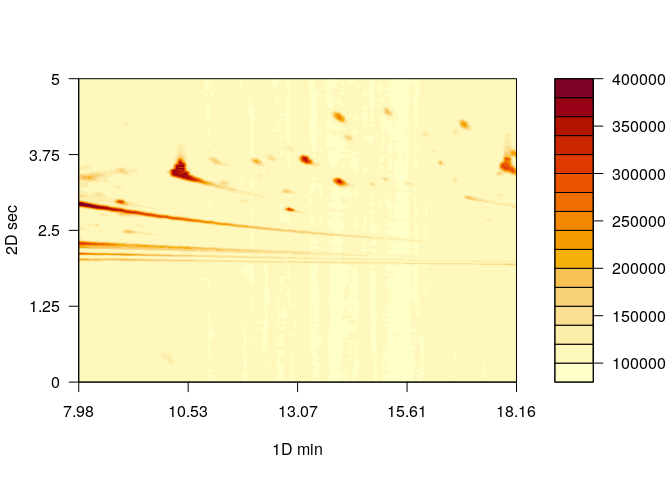The color palette of this chromatogram might not be suitable. One of the most popular color palette employed in chromatogram visualization comes from MATLAB. The colorRamps package includes two palettes to overcome this issue, the matlab.like and matlab.like2 functions.

Before to change the chromatogram color palette, we would like to make emphasis that the back-end function employed in this plot, is the filled.contour function. To change the palette, we have to provide the function that creates the palette.

library(colorRamps)
plot(MTBLS08, type = "f", color.palette = matlab.like,
main =  "matlab.like")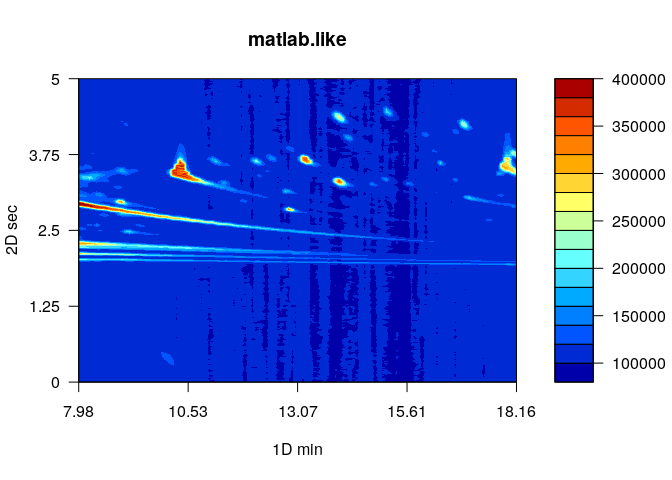plot(MTBLS08, type = "f", color.palette = matlab.like2,
main =  "matlab.like2")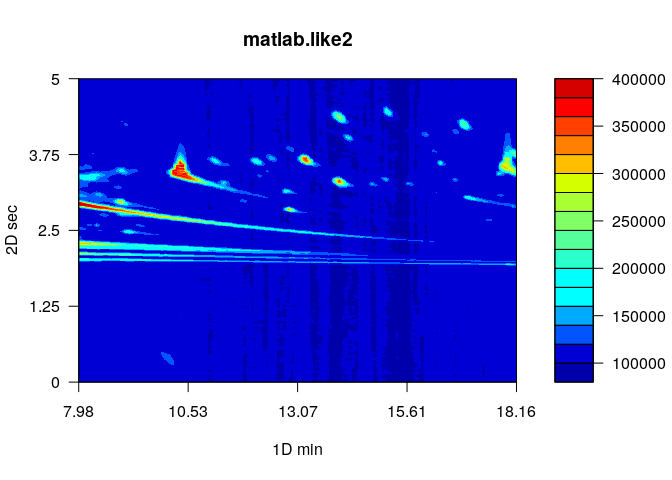We spot some similarities between this two palettes. First of all, the palette button and upper limits are very similar. Even though, the matlab.like2 function has more color breaks, what in this case, makes the chromatogram to look better. For example, in the matlab.like palette the variation of lower signals intensities is obscured by a blue degradation. On the other hand, the matlab.like palette highlight the background variation. Moreover, the color palette is highly dependent of the chromatogram as we are going to detail in the following sections.

The MS detector comes in some flavors, which two of the most common mass analyzers are Time of flight and Quadrupole. They are very different about how they work. For example, TOF mas analyzer can acquire spectra in high resolution meanwhile the quadrupole mass analyzer is not capable of. Additionally, the sampling rate achieved by TOF is higher than quadrupole. Therefore, chromatograms acquired with TOF mass analyzer are usually dimensionally higher ($$> i$$).

The previous chromatogram was acquired with TOF mass analyzer, thus TIC intensities are about $$4x10^5$$ and background intensities are about $$1x10^5$$. This is not the case of the quadrupole.

The following chromatogram is retrieved from the package article. In contrast with the previous chromatogram, the next chromatogram was acquired with a quadrupole mas analyzer.

myl_d5 <- system.file("extdata", "mylbd5.CDF", package = "RGCxGC")
myl <- read_chrom(myl_d5, mod_time = 5, sam_rate = 25, verbose = F)
plot(myl, color.palette = matlab.like2 )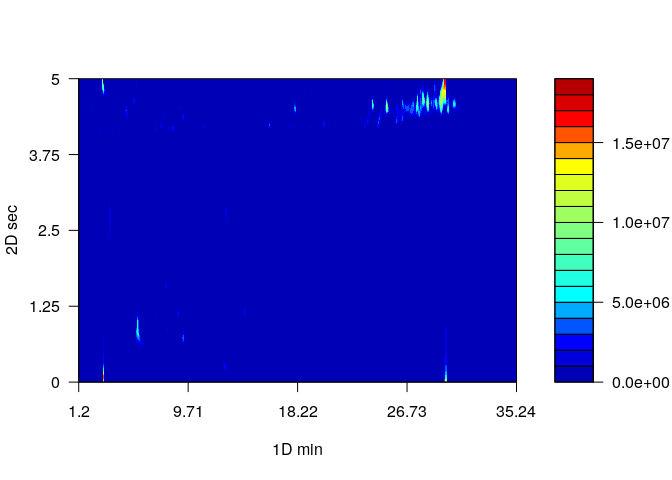Moreover, the chromatogram included in the paper is very different.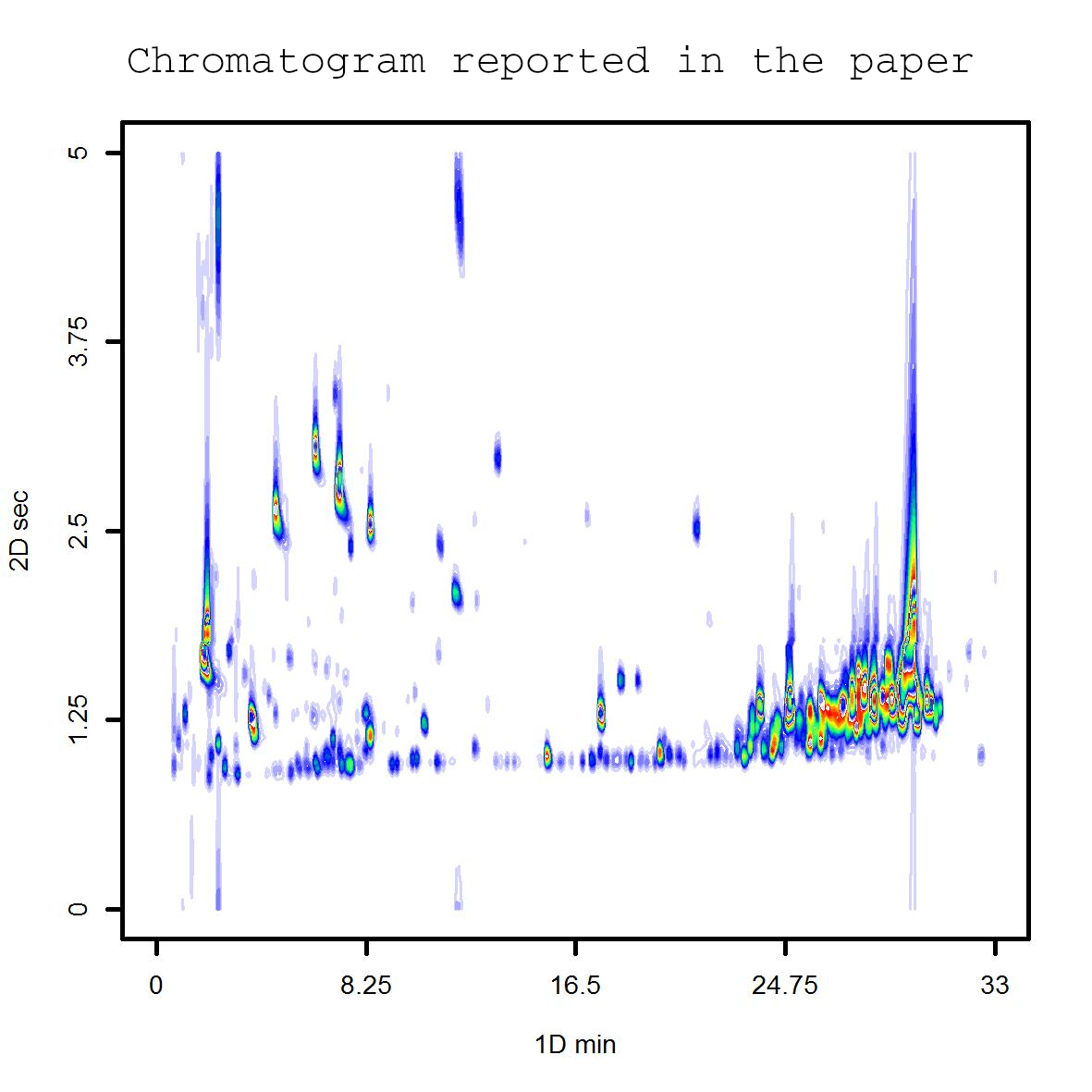The first difference is the number of features presented in both chromatograms. In the second chromatogram there are much more features. Additionally, the peaks are traslocated and they are not in the same retention time in the second dimension.

We are going to address this issues in four steps:

• Chromatogram dephasing
• Changing the type of the plot
• Creating a personalized color palette
• Changing the number of levels (breaks)

## 4.1 Chromatogram dephasing

In two-dimensional chromartography, the modulation time may lead a peak partition. It is because the modulator does not inject the total peak volume in the modulation $$(i)$$, and reinjects the remaining peak volume in the next modulation $$(i+1)$$. The first chromatogram that we plot in the section 4 presents this challenge. Therefore, in order to improve the chromatogram visualization and interpretation, the chromatogram is scrolled in the second dimension.

myl_deph <- dephase_chrom(myl, rel_dephase = 65)
plot(myl_deph, color.palette = matlab.like2 )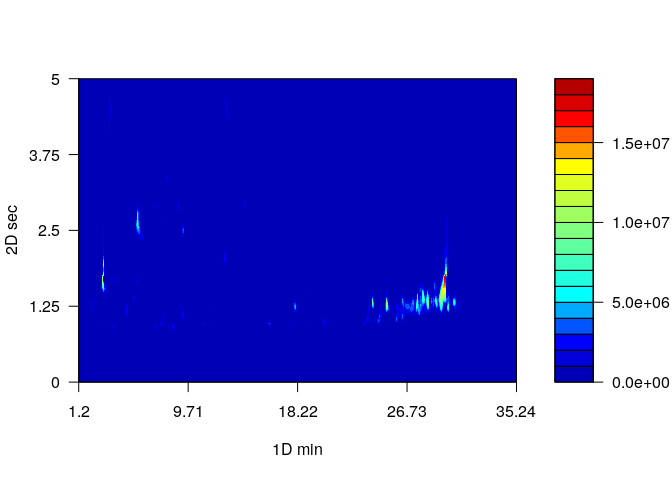The dephase_chrom scrolls the chromatogram by a given scrolling percentage. In this example, the second retention time has been scrolled 75%. We notice that the higher intensities are now in the same retention times.

## 4.2 Changing the type of plot

As aforementioned, the default plot type is the filled contour. Even though, compound concentrations is usually uneven in untargeted analysis. Therefore compounds in a low concentration are not going to be displayed with the current approach. In this case, we can opt for the contour plot. The major difference between these two types of plots is how you provide colors. In this case, you have to specify the colors, and not the function that creates those colors, and you have to specify type = "c".

plot(myl_deph, type = "c",col = matlab.like2(10) )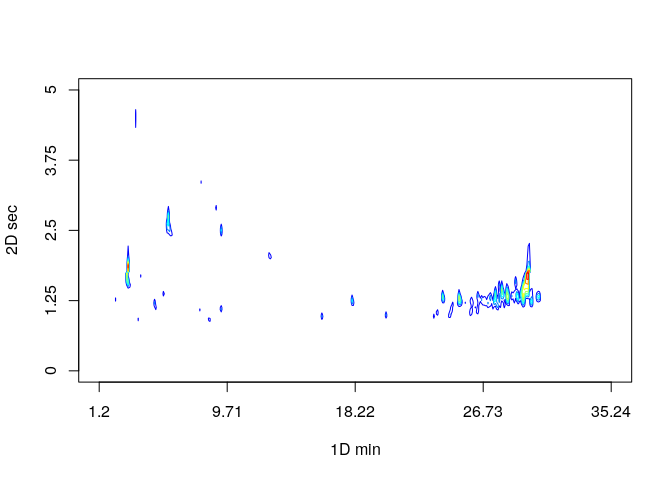We start noticing that more features came out. This is because low signals were obscured by the background signal.

## 4.3 Creating a personalized color palette

Although the matlab.like and matlab.like2 functions provides a fast solution for colors, other purposes may need other colors. Thus, we are going to explain how to create a personalized color palette.

Basically, the colorRampPalette function will be use. This function create a color palette function with the provided colors. The provided colors will be used as breaks.

my_palette <- colorRampPalette(rev(c("red","yellow","springgreen",
"blue", "white")))
plot(myl_deph, type = "c", col = my_palette(10) )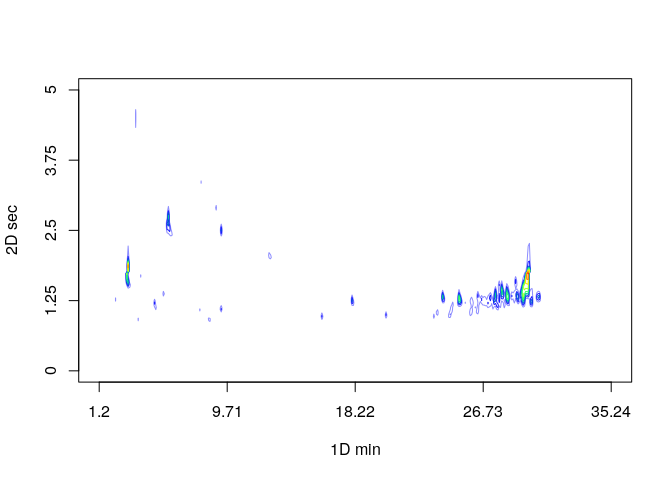With the customized my_palette function the chromatogram visualization was improved. We are almost done but more low concentration compounds are till obscured.

## 4.4 Changing the number of levels

The number of levels is a critical argument to take into consideration to create an effective chromatogram visualization. This argument affect in how many breaks the signal will be splitted. For example, if nlevels = 10 the total TIC signal will be splitted in 10 equally spaced breaks. If you set this argument to a greater value, the total TIC signal will be splitted in more breaks, which enhance the feature visualization.

plot(myl_deph, type = "c", col = my_palette(35), nlevels = 100 )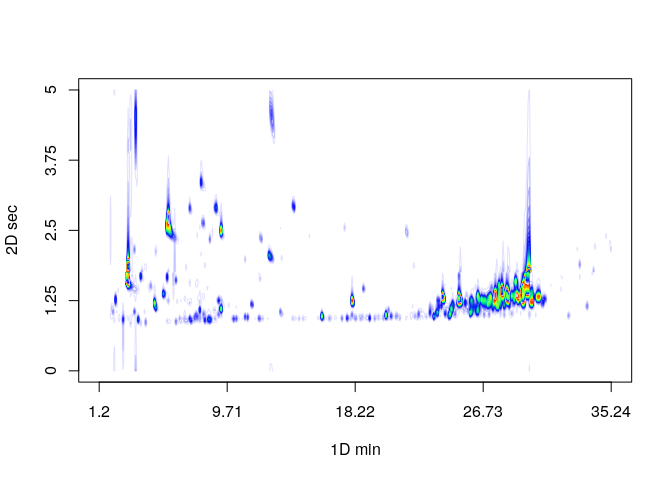As we notice, now we have a similar chromatogram with the reported in the paper.

Tip: in this case of plots, the number of levels and the number of colors should be the equal for low levels (nlevels < 50). On the other hand, for nlevels > 50, nlevels should be twice or three times the number of colors.